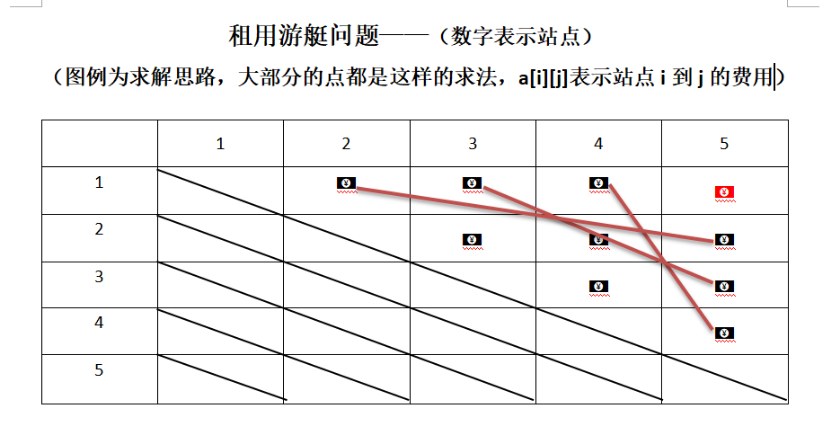# 算法第三章作业

1. 你对动态规划算法的理解
2. 分别列出编程题1、2的递归方程
3. 说明结对编程情况

1.你对动态规划算法的理解

2.分别列出编程题1、2的递归方程

（1） 求单调递增最长子序列 （时间复杂度为 O(n2)）

//没有使用递归

//将数字存于a[]中

for (int i = 1; i < n; i++){

for (int j = 0; j < i; j++)

if (a[j] < a[i]&& b[j]>b[i] - 1)

b[i] = b[j] + 1;

}

int t = b;

for (int  k = 0; k < n; k++)

{

if (b[k] > t)

t = b[k];

}

cout<<t;

（2） 租用游艇问题

t=a[i][k]+a[k][j];（ k=j-1; k>=i+1 ）

①a[i][j]=max{ a[i][j], t } ( j!=i+1 && i>=1 && j<=n )

②a[i][j]=a[i][j] ( j==i+1 )3.说明结对编程情况

posted @ 2018-11-11 20:35  树下...听雨  阅读(100)  评论(0编辑  收藏  举报